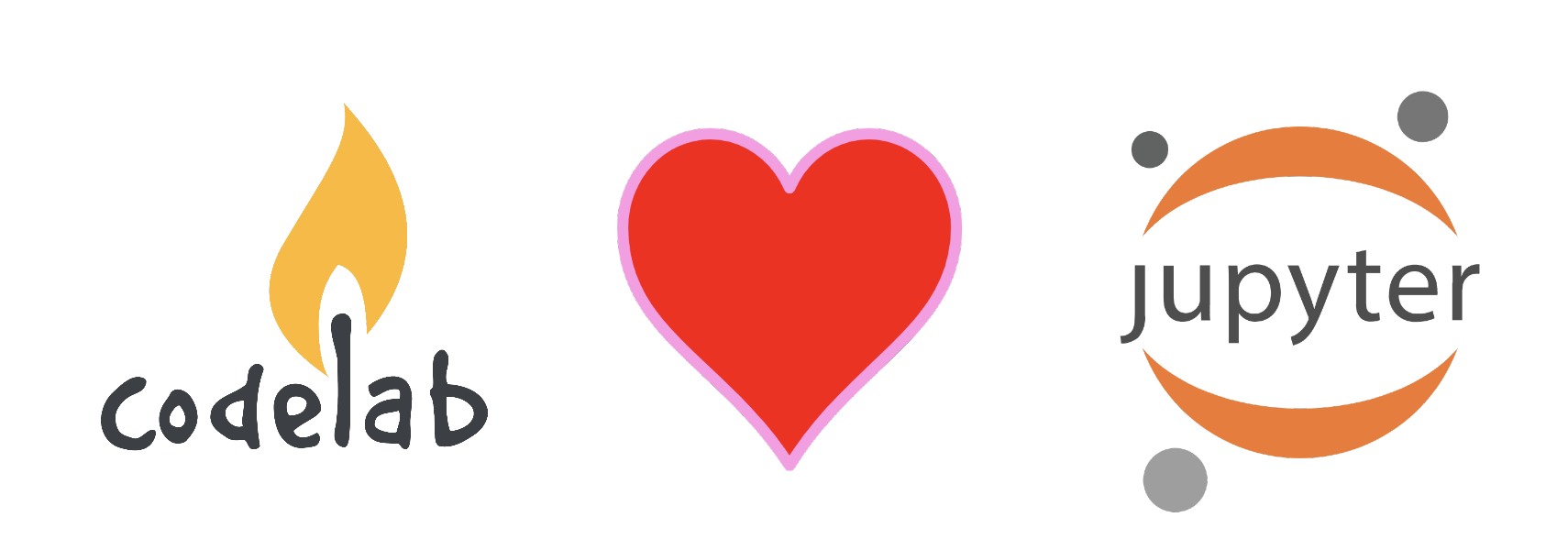# demo

 `````` 1 2 3 4 5 6 7 8 9 10 11 12 13 14 15 16 17 18 19 20 21 22 `````` ``````from codelab_adapter_client import SimpleNode node = SimpleNode() node.simple_publish("开启音乐") node.simple_publish("下一个造型") import time for i in range(10): time.sleep(0.2) node.simple_publish("落下礼花") # 完整演奏 import time node.simple_publish("开启音乐") time.sleep(0.2) for i in range(30): time.sleep(0.2) node.simple_publish("下一个造型") time.sleep(0.2) for i in range(5): node.simple_publish("落下礼花")``````

# 更多的想象空间

Jupyter是目前最理想Python/数据分析/人工智能的编程教育环境。关于Jupyter在教育上的意义可以参考使用IPython Notebook来学习编程

CodeLab Adapter 1.0致力于将万物带入Scratch，实现`education as life`的目标。CodeLab Adapter 2.0则将万物带入到Python教育中。物联网、人工智能、开源硬件，你现在可以使用Python在Jupyter中与这些东西交互。

### 机器人教学

 `````` 1 2 3 4 5 6 7 8 9 10 11 12 13 14 15 16 17 18 19 20 21 22 23 24 25 26 27 28 29 `````` ``````from codelab_adapter_client import AdapterNode from codelab_adapter_client.topic import JUPYTER_TOPIC class MyRobotNode(AdapterNode): def __init__(self): super().__init__() self.EXTENSION_ID = "eim/vector" self.message = {"topic": JUPYTER_TOPIC, "payload": {"content": "robot.behavior.say_text('hello')"}} def forward(self,distance,speed): self.message["payload"]["content"] = f"robot.behavior.drive_straight(anki_vector.util.distance_mm({distance}), anki_vector.util.speed_mmps({speed}))" self.publish(self.message) def turn_left(self,degrees,speed): self.message["payload"]["content"] = f"robot.behavior.turn_in_place(anki_vector.util.degrees({degrees}), speed=anki_vector.util.degrees({speed}))" self.publish(self.message) def say(self,content): self.message["payload"]["content"] = f"robot.behavior.say_text('{content}')" self.publish(self.message) robot = MyRobotNode() robot.forward(100,100) robot.turn_left(90,180) robot.say("hello") from pypinyin import lazy_pinyin pinyin = "".join(lazy_pinyin('肚子 好饿 去吃 午饭啦')) print(pinyin) robot.say(pinyin)``````

# 线上学习平台

@曾老师近期在参与一个AI线上学习平台的构建，将基于Open edX + Jupyter

Open edX提供了绝佳的学习系统，也许是目前全球最强大的学习系统，它拥有绝佳的开放性和丰富的生态。而Jupyter则是目前最理想的Python//数据分析/机器学习/AI编程工具。@曾老师希望结合两者，为学习者提供舒适而高效的学习环境，初学者不必为环境的搭建焦头烂额，目前斯坦福大学和UC伯克利都在使用Open edXJupyter作为编程教育平台。

 ``````1 2 3 `````` ``````from IPython.core.display import Javascript, display for i in range(3): display(Javascript(f"console.log('{i}') //websocket send/recv message <-> CodeLab Adapter 2.0")) ``````